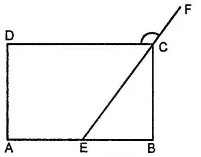Find the measure of each exterior angle of a regular polygon of

(i) 9 sides

(ii) 15 sides

Asked by Aaryan | 1 year ago |  96

##### Solution :-

(i) Sum of all exterior angles of the given polygon = 360 º

Each exterior angle of a regular polygon has the same measure.

Thus, measure of each exterior angle of a regular polygon of 9 sides

$$\frac{360^\circ}{9} = 40^\circ$$

(ii) Sum of all exterior angles of the given polygon = 360 º

Each exterior angle of a regular polygon has the same measure.

Thus, measure of each exterior angle of a regular polygon of 15 sides

$$\frac{360^\circ}{15} = 24^\circ$$

Answered by Sakshi | 1 year ago

### Related Questions

#### In the given figure, PQRS is a kite. Find the values of x and y.

In the given figure, PQRS is a kite. Find the values of x and y.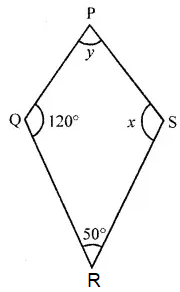#### In the given isosceles trapezium ABCD, ∠C = 102°. Find all the remaining angles of the trapezium.

In the given isosceles trapezium ABCD, ∠C = 102°. Find all the remaining angles of the trapezium.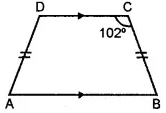#### In the given figure, ABCD is a rhombus and ∠ABD = 50°. Find :

In the given figure, ABCD is a rhombus and ∠ABD = 50°. Find :

(i) ∠CAB

(ii) ∠BCD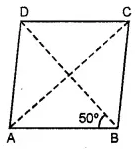#### In the given figure, ABCD is a rectangle and diagonals intersect at O. If ∠AOB = 118°, find

In the given figure, ABCD is a rectangle and diagonals intersect at O. If ∠AOB = 118°, find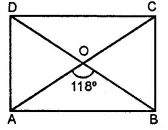(i) ∠ABO

(iii) ∠OCB